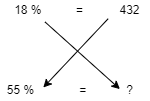# Bosch aptitude questions

If you’re looking to apply to Bosch and ace the job test, you need to be prepared for some tough aptitude questions. Bosch tests its applicantson several skills, including verbal, numerical, and logical reasoning. To get ahead of the competition, practice solving aptitude questions related to these topics. You can find aptitude question sets here or in preparation books to make sure you are ready before you sit for the test. Download the Aptitude questions and answers repeated on the Bosch Aptitude test provided below to get your dream job.

1. A pen was sold for Rs.20 with a profit 20%. If it were sold for Rs.17.66,find the approximate percentage of profit or loss?

• CP =100% , Profit = 20% $\Rightarrow$ SP = 120%

120% = 20

100% = $x$

$x\times 120 = 20\times 100$

$x$ = $\frac{100}{6}$ = 16.66

• If it were sold for Rs.17.66, there will be a profit of Rs.1.

Profit % = $\frac{loss}{CP}\times 100$

= $\frac{1}{16.66}\times100$

= 6.002% $\approx$ 6%.

1. Six years ago the ratio between the ages of Arjun and Deepthi is $6 : 5$. After $4$ years the ratio will be $11:10$ . What is the age of Deepthi at present

• Let Arjun's and Deepthi's age $6$ years before be $6x$ and $5x$ respectively.
• Then $\frac{(6x+6)+4}{(5x+6)+4}=\frac{11}{10}$
$10(6x+10)=11(5x+10)$
$5x=10$ $x=2$
• Deepthi's present age $=5x+6=16$
1. The value of $\sqrt{\frac{0.16}{0.4}}$ is = ?

• Given $\sqrt{\frac{0.16}{0.4}}$,

= $\sqrt{\frac{0.16}{0.4}}$

= $\sqrt{\frac{0.16}{0.40}}$

• Numerator and Denominator multiplied by 100

= $\sqrt{\frac{16}{40}}$

= $\sqrt{\frac{4}{10}}$

= $\sqrt{{0.4}}$

= 0.63

1. In a class boys and girls are in the ratio 5 : 6. Among the boys, the ratio of mathematics to physics students is 2 : 1. If the ratio of mathematics and physics students in the entire class is 4 : 3. What percentage of class comprises boys studying mathematics?

• Let boys and girls in the class $5x$ and $6x$ respectively.
• ∴ Total Students $= 5x+6x=11x$
• Boy students of mathematics $= \frac{2}{3}\times 5x= \frac{10}{3} x$
• Boy students of Physics $= \frac{1}{3} \times 5x= \frac{5}{3}x$
• Percentage of boy's studying in mathematics $=\displaystyle \frac{{ \frac{10}{3}}x}{11x}\times 100= 30.30 \%$
1. If the difference between 72% and 54% of a number is 432 then what is 55% of that number?

• Number $= x$

• $x \times \frac{72}{100}- x \times \frac{54}{100}=432$ <br> $x \times \frac{18}{100}=432$ <br> $x= \frac{43200}{18}=2400$ <br>

• 55 % of the number = $2400 \times \frac{55}{100}= 24 \times 55=1320$

• Easy trick

• 72% - 54% = 18%

18% $\Rightarrow$432<br> 55% $\Rightarrow$?

•= $\frac{55 \times432}{18}$ = 1320